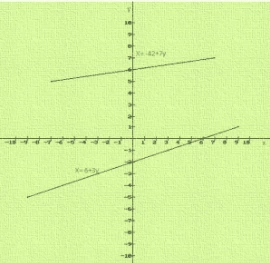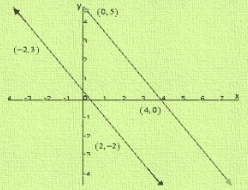# RD Sharma Class 10 and Chapter Pair Of Linear Equations In Two Variables Maths Solutions

## Maths Class 10 and Chapter Pair Of Linear Equations In Two Variables RD Sharma SolutionsFilter
Filters :
CLASSES
•
•
•
•
•
•
•
CHAPTERS
•
• 6 More

### RD SHARMA Class 10 | PAIR OF LINEAR EQUATIONS IN TWO VARIABLES

Aa n dB each have certain number of oranges. A says to B , if you give me 10 of your oranges, I will have twice the number of oranges left with you. B replies, if you give me 10 of your oranges, I will have the same number of oranges as left with you. Find the number of oranges with A and B separately.### RD SHARMA Class 10 | PAIR OF LINEAR EQUATIONS IN TWO VARIABLES

Find the values of k for which the system 2x+k y=1 3x-5y=7 will have (i) a unique solution, and (ii) no solution. Is there is a value of k for which the system has infinitely many solutions?This chapter will cover the graphical method of solutions of a pair of linear equations. A linear equation is a pattern of numbers that are increasing or decreasing by the same amount on every step of the way. Linear equations are the basic kind of algebraic function. Students can practice different exercises which have been provided by Doubtnut. It helps the students to get an accurate idea on how to work on it in the best way that would never lead to getting confused at all. So, by getting the right knowledge, it proves to be of much use to them.

The NCERT books have been used by the CBSE in their syllabus. However, it has come to notice that these books fail to provide an in-depth analysis of different topics required to excel in board exams. In this case, RD Sharma books prove to be one of the best and authentic books for Math for the students. RD Sharma books have multiple solved examples and unsolved exercises. However, even with the help of solved examples, students find it challenging to solve unsolved exercises. So, it is important to get the solutions of unsolved exercises for effective learning and practice of math.

Doubtnut is an online tutorial platform providing solutions to all the problems included in RD Sharma book. The solutions are worked out in detail through the means of pre-recorded videos, thus making it a perfect online textbook solutions platform. The video solutions are presented in simple language for ease in understanding the concepts. The solutions are provided to students at zero cost. They can access it without paying any money for it. So, students find it quite flexible where they can access it from any place and at any time. Also, lots of exercises and examples have been included to enable the students to get the whole idea on how to solve the different questions effortlessly. With the best Class 10 Maths RD Sharma Solutions, it becomes possible to get the right solutions.

## Topics and subtopics of RD Sharma class 10 Chapter 3 Pair of Linear Equations in Two Variables

### Introduction

Do you know that it is possible to find different solutions for a pair of equations. Well, it is important to note that there are lots of different ways to work on these. Here, we will come to know how to work on it.

### Pair of Linear Equations in Two Variables

In Class 9, we studied the following examples of linear equations in two variables.

2x + 4y = 6

x – 3y – 2 = 0

and  x – 0y = 2. i.e. x=2

An equation that can be set in the form of ax + by + c = 0,

a, b and c are real numbers, and also a and b are non-zero real numbers, is termed as a linear equation in two variables ‘x’ and ‘y.’

If we substitute x =1 and y =1 in the left hand side (LHS) of the equation  2x + 3y = 5. We get LHS = 2(1) + 3(1) = 2 + 3 +5

So, you can find that it happens to be equal to the RHS of the equation.

So, x = 1 and y = 1 is a solution of the equation 2x + 3y = 5.

Now if we substitute x = 1 and y = 5 in the equation 2x + 3y = 5. Then,

LHS = 2(1) + 3(5) = 2 + 15 = 17 which is not equal to the RHS.

Therefore, x = 1 and y = 5 is not a solution of the equation.

Let us have a look at an example below:

Exercise:

Rahul tells his daughter, ”Seven years ago, I was seven times as old as you were then. He also said, “I shall be three times as old as you will be three years from now.” Represent this situation algebraically and graphically.

Solution:

Let the present age of Rahul and his daughter be x and y respectively. Seven years ago,

Age of Rahul = x – 7

Age of his daughter = y – 7

According to the given condition,

x – 7 =7 (y – 7) = x – 7y = -42

Three years from hence,

x + 3 = 3 (y + 3) = x – 3y = 6

Thus the given Solution: can be algebraically represented as

= x – 7y = -42 …………………………….. (i)

= x – 3y = 6 ……………………………… (ii)From equation (i)

 X Y -7 5 0 6 7 7

x = -42 + 7y

x = 6 + 3y From equation (ii)

 X Y -7 5 0 6 7 7

So, now let’s plot it in the graph### Graphical Method of Solution of a Pair of Linear Equations

When a pair of the line is represented by the behavior of the lines its can be summarized in two variables as well as the existence of the solutions as follows:

A) There may be an intersection of the line in a single point. So, here the pair of equations has a unique solution.
B) Some lines may be coincident. Here the equations have infinitely many solutions.

C) There may be parallel lines. In this case, the equations do not have any solutions.

Let’s have a look at the exercise below:

Exercise: Lucy is walking along a path joining (-2,3)and (2,-2), while Saurav is walking along the path joining (0,5) and (4,0). Represent this situation graphically.

Solution: It is given that Lucy is walking along the path joining (-2,3) and (2,-2), while Saurav is walking along the path joining (0,5) and (4,0)### Algebraic Methods of Solving a Pair of Linear Equations

It should be noted that there are several algebraic methods.  It includes Substitution method, Elimination method, Cross-multiplication method,  In order to get the right idea on the algebraic methods of solving a pair of linear equations, we will have a look at the exercise below:

Exercise:

Sheela went to a bank to withdraw Rs. 2000. She asked the cashier to give her Rs. 50 and Rs. 100 notes only. Here Sheela received 25 notes in all. Can you find out how many notes Rs. 50 and Rs. 100 she received?

Solution:

Let the number of notes of Rs. 50 = x

Let the number of notes of Rs. 100 = y

According to the question,

Case 1:

Suppose Sheela asks for Rs. 50 and Rs. 100 notes only, we get the equation,

50x + 100y = 2000

x + 2y = 40 ——- (i)

Case 2:

Suppose Sheela got 25 notes in all, we get the equation,

x + y = 25 ——- (ii)

Subtracting the equation (ii) from (i) we get

y = 15

Substituting the value of y = 15 in equation (ii), we get

x + y = 25

x + 15 = 25

x = 25 – 15

x = 10

Hence, x = 10 and y = 15

Therefore, Sheela has got 10 notes of Rs. 50 and 15 notes of Rs. 100 each

### Equations Reducible to a Pair of Linear Equations in Two Variables

There are pairs of equations which is not found to be linear but it can be reduced to linear form with the help of some suitable substitutions. Well, here we will solve one exercise.

Exercise:

The students of a class are required to stand in rows. There would be 1 row less if 3 students are extra in a row. There would be 2 rows more in case 3 students are less in arrow. Can you find out the number of students in the class?

Solution:

Let the number of students be x

Let the number of rows be y.

Here the number of students in each row = x/y

According to the question,

If three students are extra in each row, then there is one row less that is when each row has =

x/y+3 students.

The number of students in each row=

x/y+3 students

The number of rows = (y – 1)

We know that the total number of students = number of rows x number of student in each row

x=(x/y+3)(y−1)

x=(x+3y−x/y−3)

0=−x/y+x−x+3y−3

0=−xy+3y−3          —— (i)

If there are three students which are less in each row then there would be 2 more rows that are when each row has

(x/y−3)(y+2)

Since the total number of students = number of rows x number of students in each row

x= (x/y−3)(y+2)

x=x−3y + 2y/z−6

0=2x/y+x−x−3y−6

0=2x/y−3y−6   —- (ii)

Substituting,

x/y=u

in (i) and (ii) we get

-u + 3y – 3 = 0 ——- (iii)

2u – 3y – 6 = 0 —— (iv)

Now, on adding (iii) and (iv) we get

u – 9 = 0

u = 9

Substituting u = 9 in equation (iii) we get

-u + 3y – 3 = 0

-9 + 3y – 3 = 0

3y – 12 = 0

3y = 12

y = 12/3 = 4

Now,

X/Y=9

X/4=9

x = 36

Therefore, the number of students in the class is 36.

## Benefits of learning from Doubtnut

Doubtnut has helped students to gather all the right information on how to come up with an accurate solution. They get to know all the proper technique on how to attempt even the most difficult questions without any worry at all. Therefore, it leads to feeling themselves quite confident, and this leads to like the subject in the best manner. Some of the other advantages for the students are as follows:

• Provides to the point answers: The students learn from to-the-point answers that prove to be much use to them. Students can find that the process of getting the right idea on a particular topic has become quite more comfortable as well. So, it proves to be of much use to them where they do not take much time to solve a particular question.
• Becomes flexible for the students: Interestingly, students can download the entire study materials free of cost. By downloading it in the pdf format, it becomes possible to access it anywhere without any problem at all. So, it truly helps them to save a lot of time and money. Once they make the best decision to opt for the video tutorials, it becomes quite easy to get the best concept on how to attempt it without any difficulties at all.
• Students get the best support: The team of experts of Doubtnut always promises to provide the students with the best support. Here, students can find it to be quite useful to get all their queries cleared. So, if a student is looking forward to achieving the best result, then these online study materials can surely act as the best weapon to equip the ultimate knowledge.